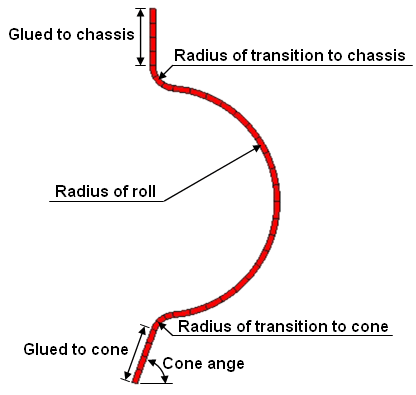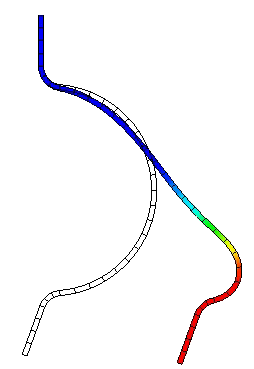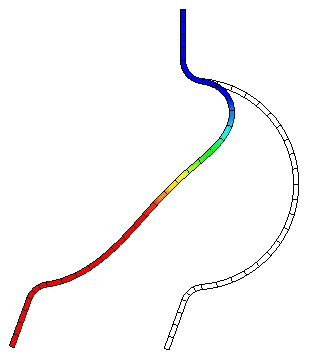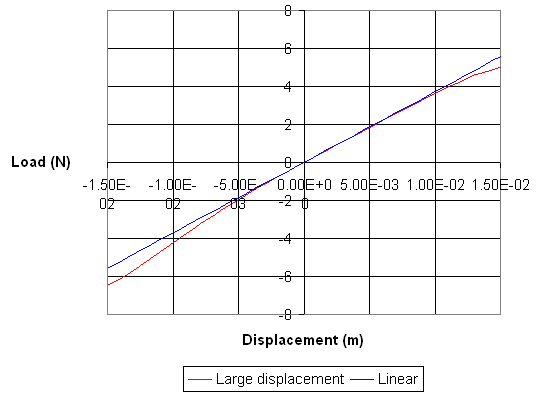## PAFEC VibroAcoustics - Large displacement analysis of a surround

 A surround can undergo large displacements, particularly at low frequencies. For large rotations the linear strain measure is not accurate. Using a large displacement analysis the load-displacement curve can be computed. This non-linearity is a potential cause of distortion.Finite element model of a loudspeaker surroundMaximum positive deformationMaximum negative deformationAt small displacements the strains and rotations are assumed to be infinitesimal and the load displacement response is linear (blue line). When the deformation includes large rotations, the assumptions of linear theory are no longer appropriate, and it becomes necessary to choose a more accurate strain measures (objective). The large displacement solution shown opposite is based on a total Lagrangian formulation using the logarithmic strain.